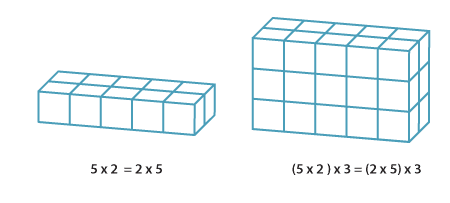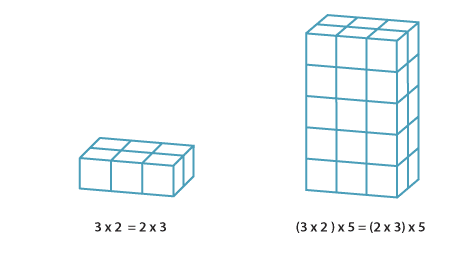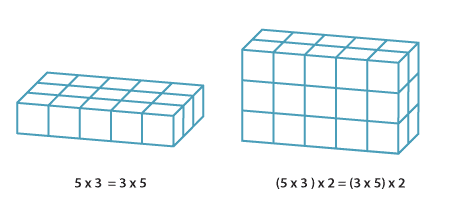### The any-order property of multiplication

A list of whole numbers can be multiplied two at a time in any order to give the same result.

Multiplying three whole numbers corresponds geometrically to calculating the number of unit cubes in (or volume of) a rectangular prism. The any-order property of multiplication means that we can calculate this volume by multiplying the lengths of the sides in any order. The order of the calculation corresponds to slicing the volume up in different ways.Detailed descriptionsDetailed descriptionsDetailed descriptions